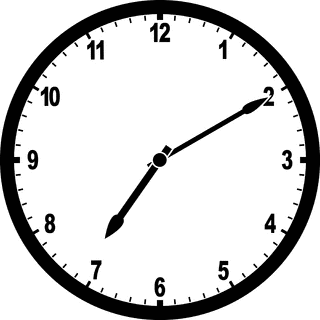Geometry

# Degrees and Radians

Convert the angle $\frac{4\pi}{3}$ radians into degrees.

Jacky drew an angle of radian measure $\frac{ 5\pi }{3}$. How many degrees is that equivalent to?The hands of a clock show 7:10. What is the measure, in radians, of the smaller angle formed between the hour and minute hands?

Round your answer to one decimal place.

Two connected gears are rotating. The smaller gear has a radius of $r_1 = 6 \text{ cm}$ and the larger gear's radius is $r_2 = 42 \text{ cm}.$ (The speed of the gears are the same as they are connected.) What is the angle in radian through which the larger gear has rotated when the smaller gear has made one complete rotation?

Which of the following is NOT true?

×